### Waves and Light

In the last quiz, we explored basic wave anatomy. In this quiz, we will build on this to learn about wave frequency and velocity. In our daily lives, frequency refers to how often something happens. For example, if you go to the grocery store every Monday, the frequency of your grocery shopping is once a week. If you instead went grocery shopping every Monday and Friday, your frequency would increase to twice a week. In other words, when more of something happens within a certain time frame, the frequency increases!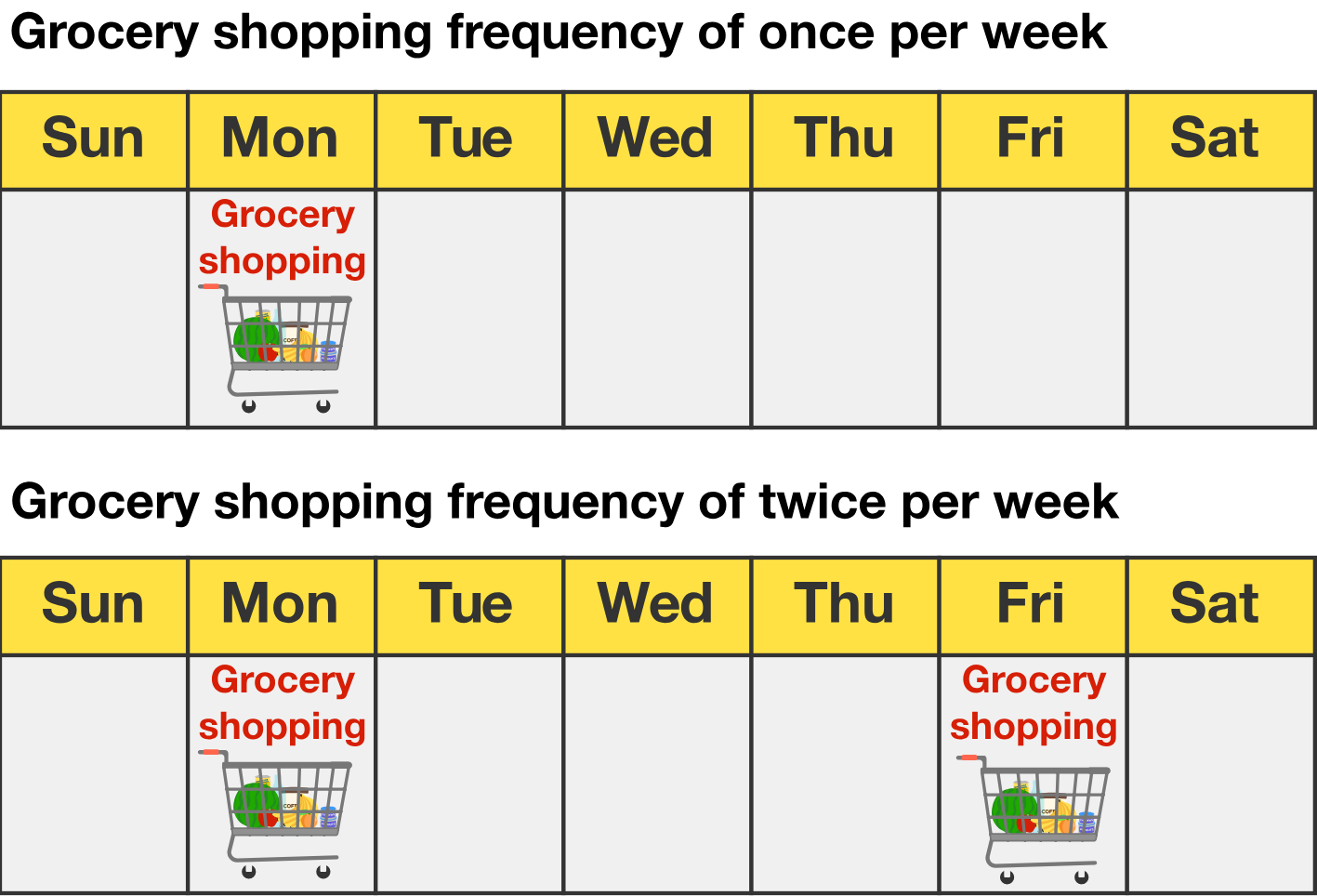# Wave Frequency and Velocity

Frequency is a familiar concept that we use every day, and it’s not much different in physics! A wave’s frequency $f$ is the number of complete wavelengths that pass a point in a certain amount of time. It is most often represented in units of per second $(\si{\second}^{-1}$), or hertz $(\si{\hertz}).$

While you'll recall from the previous quiz that the period tells us the time it takes for one wave cycle to complete, the frequency can be used to describe how many wavelengths pass a point within a certain time interval. If a complete wavelength passes a point every second, its frequency is $\dfrac{\textrm{1 wavelength}} {\si{\second}}.$ This can be written simply as $\SI{1}{\hertz}.$ If $320$ wavelengths pass a point in a second, the frequency is $\SI{320}{\hertz}.$ When more wavelengths pass a point per unit time, we say that the frequency of the wave is higher. Which of the following traveling waves has the highest frequency?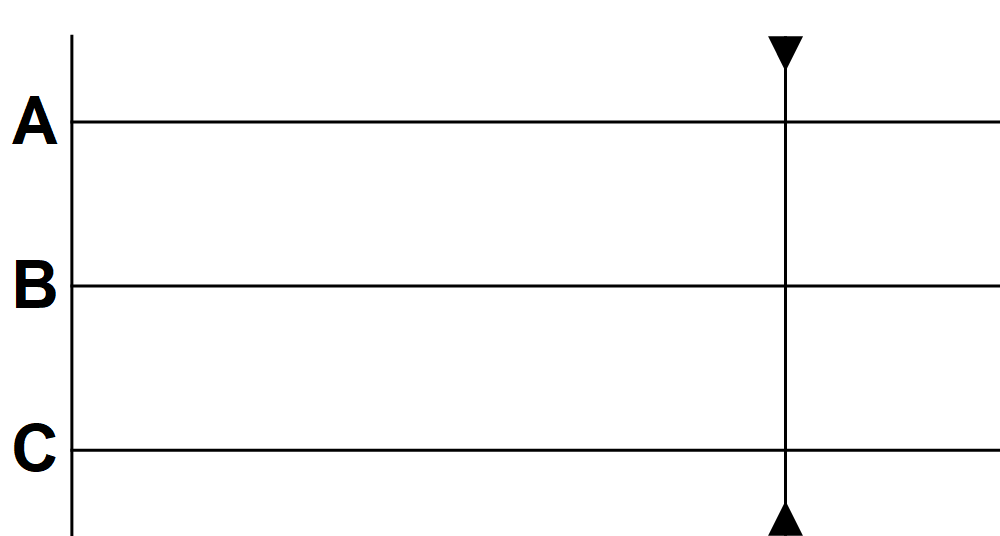# Wave Frequency and Velocity

There is an important relationship between velocity, wavelength, and frequency. We’ll uncover this relationship over the course of this quiz, starting with an intuitive example. Suppose that there are two lanes on a highway: one lane has only large trucks and the other has only cars. If both lanes move at the exact same speed, will more cars or trucks pass the cones (orange line) after a given amount of time?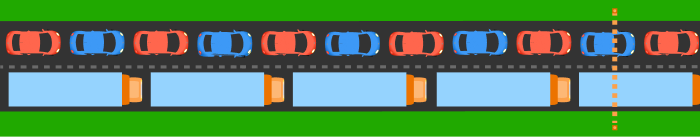# Wave Frequency and Velocity

Like the cars and trucks, these traveling waves are propagating at the same velocity. What can we conclude about the relationship between wavelength and frequency by observing them?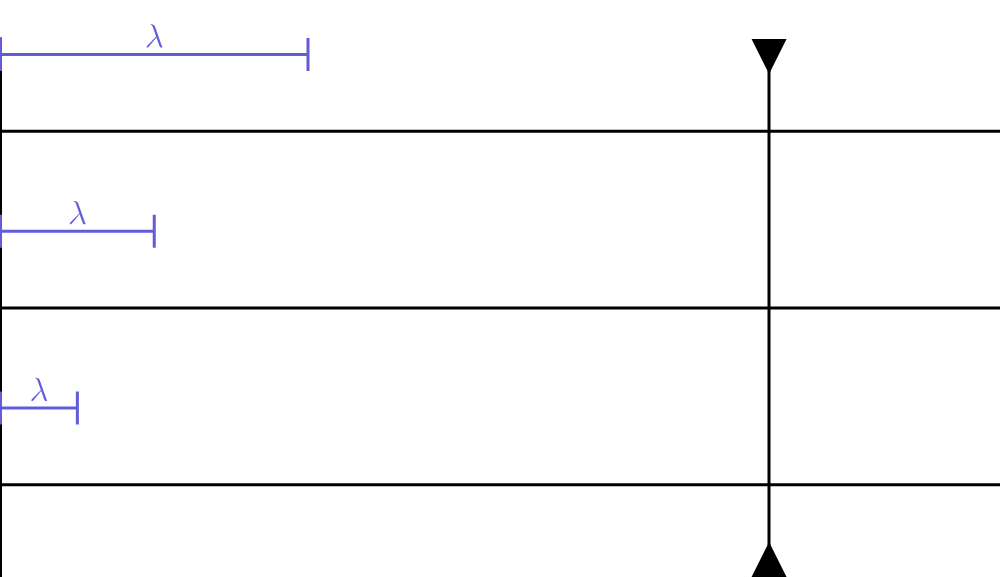# Wave Frequency and Velocity

The velocity a wave propagates at is simply referred to as wave velocity $\mathbf{v}:$ $\textrm{velocity = }\dfrac{\text{displacement}}{\textrm{change in time}}\ \ \text{ or }\ \ \mathbf{v}(t) = \dfrac{\Delta \mathbf{x}}{\Delta t}.$ How can we represent wave velocity using wavelength $\lambda$ and period $T?$

Remember: The period $T$ is the time it takes to complete one wavelength. While the wave’s period is a measure of time, wavelength is a measure of length. We could measure a period in seconds using a stopwatch, but we measure wavelength as a length in meters, centimeters, nanometers, etc.

# Wave Frequency and Velocity

In the last problem, we determined that wave velocity $\mathbf{v}$ is ${\color{#EC7300} \textrm{v} = \frac{\lambda}{T}}.$ We also know that frequency $f$ is the number of waves that pass a point per unit time and that it is represented in units of $\si{\second}^{-1}$ or hertz $(\si{\hertz}).$ But if instead of seconds, we say that amount of time is the period $T$, the frequency is then equivalent to the inverse of the period $T:$ $f = \frac{1}{T}.$ We can replace the period $T$ in the formula for wave velocity with frequency $\color{#EC7300}{\textrm{v} = \frac{\lambda}{T}} \color{#000000}\implies \color{#205aae}\textrm{v} = \lambda f$ to obtain a very important formula for waves: $\color{#205aae}\boxed{\textrm{v} = \lambda f}.$ This formula astonishingly relates three critical characteristics of waves. This formula confirms what we observed earlier—wavelength and frequency are inversely proportional.

# Wave Frequency and Velocity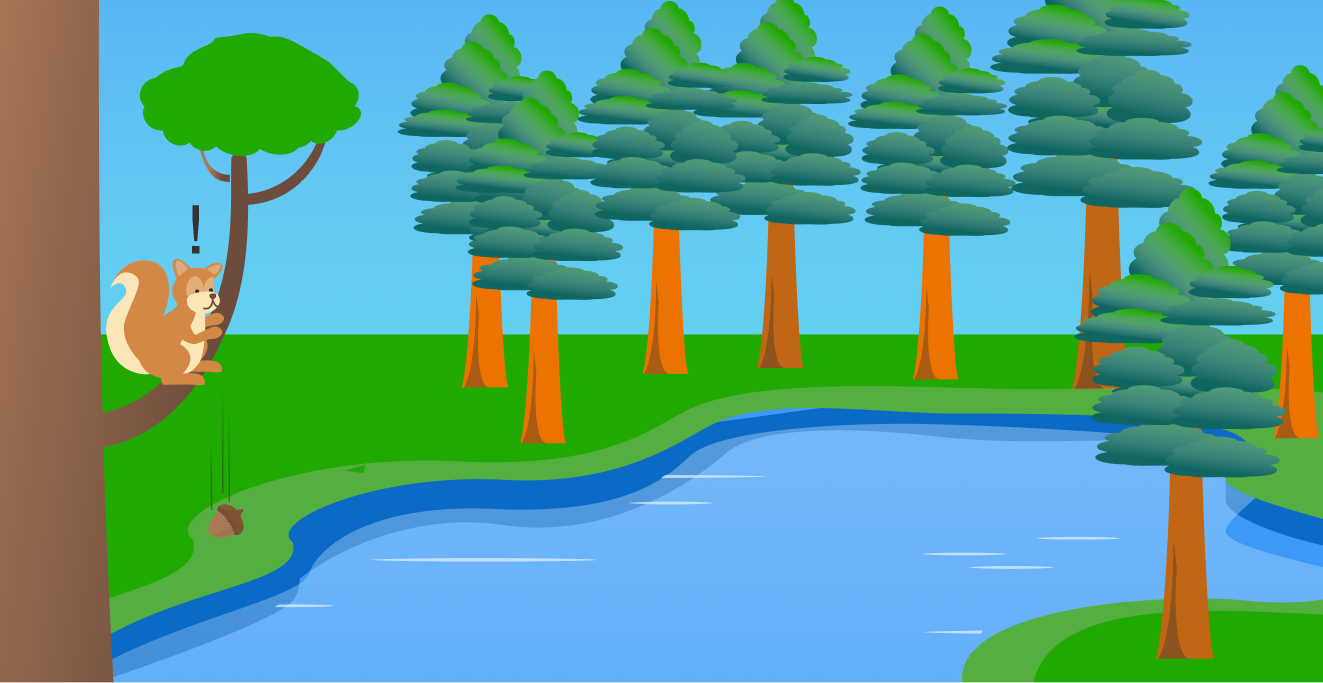Let’s put this equation to use! Say you're hiking through a forest when you see a squirrel in a tree accidentally drop its acorn. The acorn falls down into the pond below, creating ripples—concentric circles made of crests and troughs that spread out from the spot where the acorn fell in.

If the distance between the ripples is $\SI{7}{\centi\meter}$ and you see $4$ ripples hit the edge of the pond near you each second, what is the velocity of the traveling wave that's causing the ripples?

Remember: $\color{#205aae}\textrm{v} = \lambda f$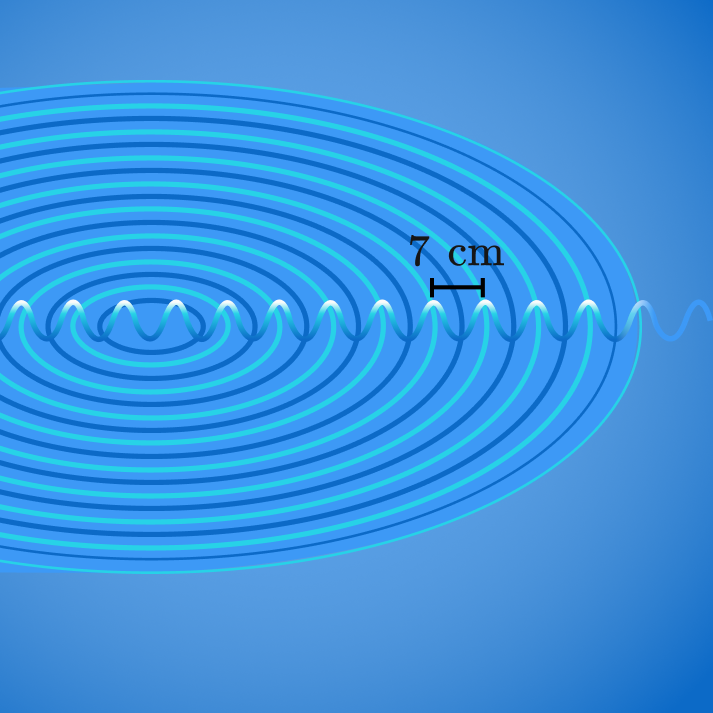# Wave Frequency and Velocity

As we will explore in the coming chapter, a wave's properties depend on its medium. This includes a wave's velocity. The speed that sound waves travel, or just the speed of sound for short, is dependent on the medium’s density and temperature. While the speed of sound in air at 20°C is ~$\SI[per-mode=symbol]{343}{\meter\per\second},$ if the temperature is 35°C, the speed of sound is ~$\SI[per-mode=symbol]{352}{\meter\per\second}!$

Human ears are generally capable of picking up frequencies between $20$ to $20,000 \si{\hertz}.$ But the hearing ranges of other animals can sometimes include frequencies significantly higher or lower than this. If a mother mouse picks up a sound wave traveling at $\SI[per-mode=symbol]{343}{\meter\per\second}$ with a wavelength of $\SI{0.858}{\centi\meter},$ can you hear it?

Hint: Determine the wave's frequency using our formula, $\color{#205aae}\textrm{v} = \lambda f.$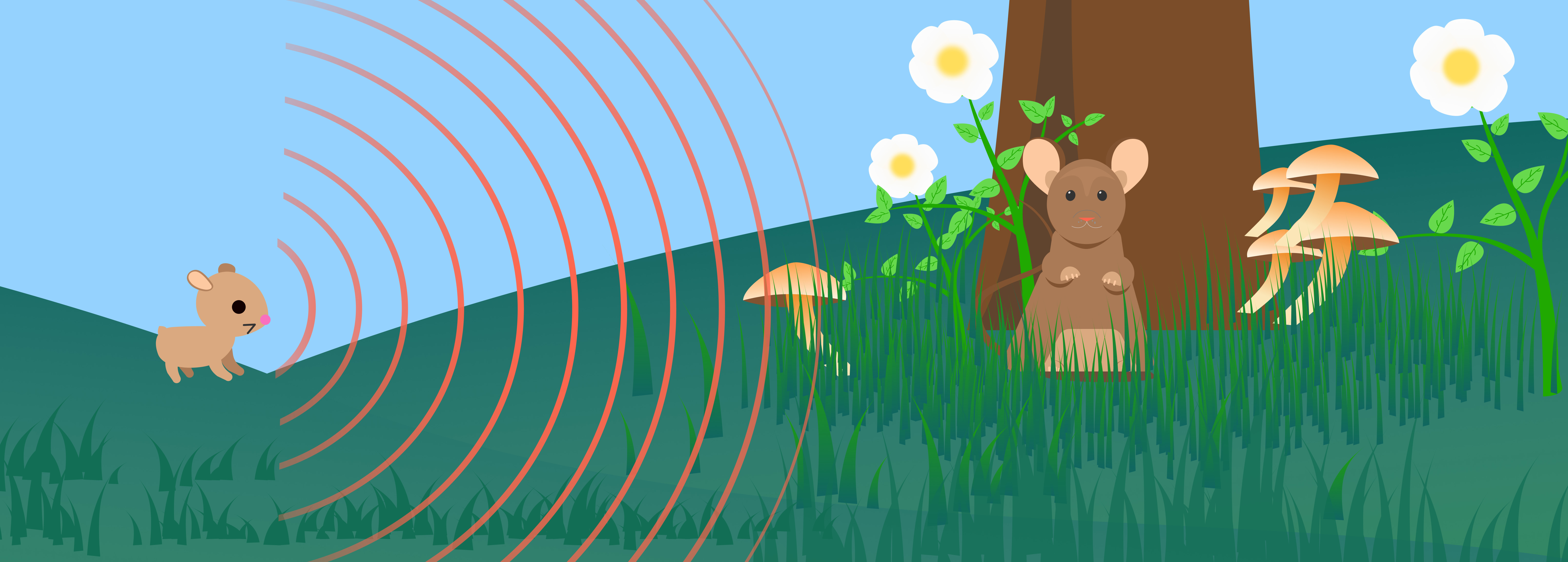# Wave Frequency and Velocity

In this quiz, we learned the following:

• Frequency $f$ is the number of waves that pass a point in a certain amount of time.
• Wave velocity $\mathbf{v}$ is the velocity that a wave propagates and is $\color{#EC7300}\textrm{v} = \dfrac{\lambda}{T} \color{#000000}\ \textrm{ or }\ {\color{#205aae}\textrm{v} = \lambda f}.$
• Therefore, as wavelength increases, frequency decreases!

In the next chapter, we’ll apply some of what we’ve learned about basic wave anatomy to explore wave behaviors like interference, reflection, and transmission, as well as how these wave behaviors result in the formation of standing waves!

# Wave Frequency and Velocity

×